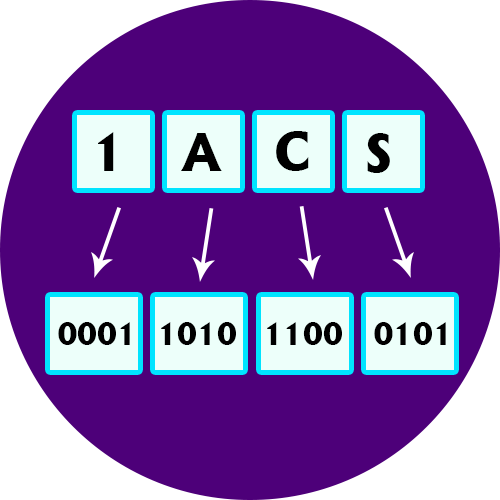﻿ Hex to Binary Converter | Hexadecimal to Binary Conversion Calculator

# Hex to Binary Converter

Use our Hex to Binary converter a free online hexadecimal calculator / conversion online tool to convert hex value into binary number system.

A Hexadecimal number system has sixteen (16) alphanumeric values from 0 to 9 and A to F. Every number (value) represents with 0,1,2,3,4,5,6, 7,8,9,A,B,C,D,E and F in this number system. The base of hexadecimal number system is 16, because it has 16 alphanumeric values. Here A is 10, B is 11, C is 12, D is 14, E is 15 and F is 16.

#### Binary Number System

A Binary number system has only two digits that are 0 and 1. Every number (value) represents with 0 and 1 in this number system. The base of binary number system is 2, because it has only two digits.

### What is Hexadecimal Number System ?

The “Hexadecimal” or simply “Hex” numbering system uses the Base of 16 system and are a popular choice for representing long binary values because their format is quite compact and much easier to understand compared to the long binary strings of 1’s and 0’s.

Being a Base-16 system, the hexadecimal numbering system therefore uses 16 (sixteen) different digits with a combination of numbers from 0 through to 15. In other words, there are 16 possible digit symbols.

The main advantage of a Hexadecimal Number is that it is very compact and by using a base of 16 means that the number of digits used to represent a given number is usually less than in binary or decimal. Also, it is quick and easy to convert between hexadecimal numbers and binary.

The main reason why we use hexadecimal numbers is because it provides a more human-friendly representation and is much easier to express binary number representations in hex than it is in any other base number system. Computers do not actually work in hex. Lets take an example, using a byte.

#### Why do Computers use Hex Number System

Computers convert binary data into the hexadecimal (hex) number system because it is much less complex than converting data into decimal numbers, and it is much easier for human beings to read hex numbers than to read binary numbers. This way, even though the actual processing and inner workings of computers use the binary system, they often display information using the hex system. Hexadecimal numerals are widely used by computer system designers and programmers because they provide a human-friendly representation of binary-coded values. Each hexadecimal digit represents four bits (binary digits), also known as a nibble (or nybble), which is half a byte.

### Standard number system conversion table

0 0000 0 0
1 0001 1 1
2 0010 2 2
3 0011 3 3
4 0100 4 4
5 0101 5 5
6 0110 6 6
7 0111 7 7
8 1000 10 8
9 1001 11 9
10 1010 12 A
11 1011 13 B
12 1100 14 C
13 1101 15 D
14 1110 16 E
15 1111 17 F### Spreading Knowledge Across the World

USA - United States of America  Canada  United Kingdom  Australia  New Zealand  South America  Brazil  Portugal  Netherland  South Africa  Ethiopia  Zambia  Singapore  Malaysia  India  China  UAE - Saudi Arabia  Qatar  Oman  Kuwait  Bahrain  Dubai  Israil  England  Scotland  Norway  Ireland  Denmark  France  Spain  Poland  and  many more....